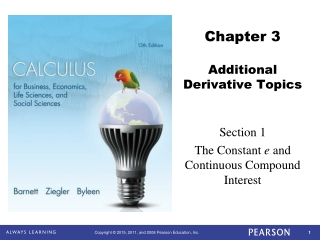DownloadDownload Presentation# Chapter 3 Additional Derivative Topics

Download Presentation## Chapter 3 Additional Derivative Topics

- - - - - - - - - - - - - - - - - - - - - - - - - - - E N D - - - - - - - - - - - - - - - - - - - - - - - - - - -
##### Presentation Transcript

1. Chapter 3Additional Derivative Topics Section 1 The Constant e and Continuous Compound Interest

2. Objectives for Section 3.1 e and Continuous Compound Interest • The student will be able to work with problems involving the irrational number e • The student will be able to solve problems involving continuous compound interest.

3. The Constant e Reminder: By definition, e = 2.718 281 828 459 … Do you remember how to find this on a calculator? e is also defined as either one of the following limits:

4. Compound Interest • Let P = principal, r = annual interest rate, t = time in years, n = number of compoundings per year, and A = amount realized at the end of the time period. • Simple Interest: A = P (1 + r) t • Compound interest: • Continuous compounding: A = P ert.

5. Compound Interest Derivation of the Continuous Compound Formula: A = P ert.

6. ExampleGenerous Grandma Your Grandma puts \$1,000 in a bank for you, at 5% interest. Calculate the amount after 20 years. Simple interest: A = 1000 (1 + 0.05•20) = \$2,000.00 Compounded annually:A = 1000 (1 + .05)20 =\$2,653.30 Compounded daily: Compounded continuously:A = 1000 e(.05)(20) = \$2,718.28

7. Example IRA • After graduating from Barnett College, Sam Spartan landed a great job with Springettsbury Manufacturing, Inc. His first year he bought a \$3,000 Roth IRA and invested it in a stock sensitive mutual fund that grows at 12% a year, compounded continuously. He plans to retire in 35 years. • What will be its value at the end of the time period? • The second year he repeated the purchase of an identical Roth IRA. What will be its value in 34 years?

8. Example (continued) • After graduating from Barnett College, Sam Spartan landed a great job with Springettsbury Manufacturing, Inc. His first year he bought a \$3,000 Roth IRA and invested it in a stock sensitive mutual fund that grows at 12% a year, compounded continuously. He plans to retire in 35 years. • What will be its value at the end of the time period? • A = Pert = 3000 e(.12)(35) =\$200,058.99 • The second year he repeated the purchase of an identical Roth IRA. What will be its value in 34 years? • \$177,436.41

9. Example Computing Growth Time How long will it take an investment of \$5,000 to grow to \$8,000 if it is invested at 5% compounded continuously?

10. Example (continued) How long will it take an investment of \$5,000 to grow to \$8,000 if it is invested at 5% compounded continuously? Solution: Use A = Pert.